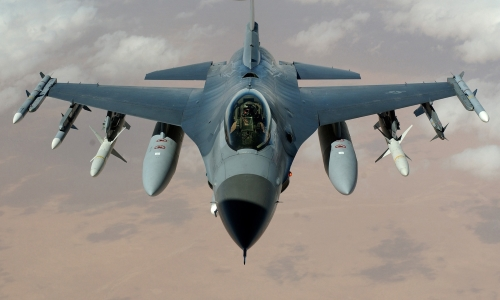# Designing an F16 fighter jetIn this tutorial, we will show how to model nonlinear aircraft dynamics in Collimator. We will use the F16 model as a case study. The F16 dynamics were firstly introduced in NASA Technical Paper No. 1538, 1979. A reduced fidelty version is written in Fortran for the F16 in Stevens et al, 2015. Here, we will build the F16 nonlinear model in Collimator and investigate how to run an open loop simulation at different operating points.

We begin by importing the following libraries:

import numpy as np
from scipy import optimize
import matplotlib.pyplot as plt
import control as ctrl
import collimator as C



## Modeling the F16 Jet

Before we start modeling, we will consider the following assumptions:

1. Rigid and symmetric aircraft.
2. The inertial reference frame is a flat and non rotating earth model.
3. Constant mass: Fuel consumption is neglected.
4. Low-fidelty aerodynamic profile as provided by Stevens et al, 2015 where the leading-edge flap dynamics is neglected.

The F16 has the following parameters

An aircraft nonlinear model consists of 15 states as illustrated in the following table:

### Equations of Motion

The 6DoF nonlinear dynamics are described as follows [Stevens et al, 2015]:

$$\begin{bmatrix} \dot{x}_E \\ \dot{y}_E \\ -\dot{h} \end{bmatrix} = \begin{bmatrix} \cos\left(\psi\right)& -\sin\left(\psi\right)& 0\\ \sin\left(\psi\right)& \cos\left(\psi\right)& 0\\ 0 & 0 & 1 \end{bmatrix} \begin{bmatrix} \cos\left(\theta\right)& 0 & \sin\left(\theta\right)\\ 0 & 1 & 0\\ -\sin\left(\theta\right) & 0 & \cos\left(\theta\right) \end{bmatrix} \begin{bmatrix} 1 & 0 & 0\\ 0 & \cos\left(\phi\right) & -\sin\left(\phi\right) \\ 0 & \sin\left(\phi\right) & \cos\left(\phi\right) \end{bmatrix} \begin{bmatrix} U\\ V\\ W \end{bmatrix}$$

2.Kinematic equation

$$\begin{bmatrix} \dot{\phi}\\ \dot{\theta}\\ \dot{\psi} \end{bmatrix} = \begin{bmatrix} 1 & \sin\left(\phi\right)\tan\left(\theta\right) & \cos\left(\phi\right)\tan\left(\theta\right)\\ 0 & \cos\left(\phi\right) & -\sin\left(\phi\right)\\ 0 & \frac{\sin\left(\phi\right)}{\cos\left(\theta\right)} & \frac{\cos\left(\phi\right)}{\cos\left(\theta\right)} \end{bmatrix} \begin{bmatrix} P\\ Q\\ R \end{bmatrix}$$

3. Force equations: $$\dot{U} = R V - Q W - g\sin\left(\theta\right) + \frac{F_x+F_{th}}{m}$$

$$\dot{V} = PW - RU + g\cos\left(\theta\right)\sin\left(\psi\right) + \frac{F_y}{m}$$$$\dot{W} = QU - PV + g\cos\left(\theta\right)\cos\left(\psi\right) + \frac{F_z}{m}$$

4. Moment equations:

$$\Gamma = I_{xx}I_{zz}-I_{xz}^2$$$$\Gamma\dot{P} = I_{zz}L + I_{xz}N - \left(I_{zz}\left(I_{zz}-I_{yy}\right)+I_{xz}^2\right)QR + I_{xz}\left(I_{xx}-I_{yy}+I_{zz}\right)PQ$$$$I_{yy}\dot{Q} = M + \left(I_{zz}-I_{xx}\right)PR - I_{xz}\left(P^2-R^2\right)$$$$\Gamma\dot{R} = I_{xx}N + I_{xz}L + \left(I_{xx}\left(I_{xx}-I_{yy}\right)+I_{xz}^2\right)PQ - I_{xz}\left(I_{xx}-I_{yy}+I_{zz}\right)QR$$

The above dynamics can be implemented in Collimator and decoupled into rotational and translational dynamics. The rotational dynamics block converts the aircraft moments into body rates and orientation Euler angles. This block is implemented as follows:

In the meantime, the translational dynamics block can be implemented as:

The overall 6-DoF equations of motion model block is modelled as follow:

### Atmosphere Model

We will use an approximation of the international standard atmospheric model as defined in [Sonneveldt, 2006].

$$T = T_0 - 0.0065h$$

$$\rho = \rho_{0}e^{-\frac{g_0}{287.05T}h}$$$$\text{Mach} = \frac{v_t}{\sqrt{1.4\times287.05T}}$$$$\bar{q} = \frac{1}{2}\rho{v_t}^2$$$$g = g_0\frac{{R_e}^2}{(R_e+h)^2}$$

where $\rho_{0} = 1.225\text{ }\frac{kg}{m^3}$ is the air density at sea level, $g_0 = 9.80665\text{ }\frac{m}{s^2}$ is the gravity acceleration at sea level, $T_0 = 288.15\text{ }K$ is the temperature at sea level, $R_e = 6371000\text{ }m$ is the mean earth radius, $\bar{q}$ is te free stream air pressure. This model is valid for an upper atmospheric ceiling of $11000\text{ }m$. We will implement the above atmospheric model as a custom block to allow reusability:

### Aerodynamics

This block is responsible for computing the aerodynamic forces $(F_x, F_y, F_z)$ and moments $(L, M, N)$ which are defined as:

$$F_x = \bar{q}SC_x\left(\alpha,\beta,\delta_e, \delta_a, \delta_r, \text{Mach}\right)$$$$F_y = \bar{q}SC_y\left(\alpha,\beta,\delta_e, \delta_a, \delta_r, \text{Mach}\right)$$$$F_z = \bar{q}SC_z\left(\alpha,\beta,\delta_e, \delta_a, \delta_r, \text{Mach}\right)$$$$L = \bar{q}BSC_l\left(\alpha,\beta,\delta_e, \delta_a, \delta_r, \text{Mach}\right)$$$$M = \bar{q}\bar{c}SC_m\left(\alpha,\beta,\delta_e, \delta_a, \delta_r, \text{Mach}\right)$$$$N = \bar{q}BSC_n\left(\alpha,\beta,\delta_e, \delta_a, \delta_r, \text{Mach}\right)$$

The $C_x, C_y, C_z, C_l, C_m, C_n$ are the aerodynamic coefficients of the aircraft which are nonlinear functions. For more details of how to calculate the coefficients, please refer to [Stevens et al, 2015]. Each one of these coefficients is defined as a lumped function of a set of underlying coeffecients such as the aerodynamic derivatives, control surfaces coefficients, etc. The aerodynamic block is modelled as in the following figure.

Here in this model, we will use a low-fidelty aerodynamic profile as provided by Stevens et al, 2015. The underlying coefficients are defined as a lookup table. For example, the aerodynamic derivates subblock is modelled as:

### Controls

The F16 model can be controlled through engine thrust and three control surfaces: elevator, ailerons, and rudder. The control actuators are all modelled as a a first-order transfer function with limits on outputs and rates. The actuator block is modelled in Collimator as the following diagram:

The control actuators data are summarized in the following table:

## Running the Simulation

### Trimming the Model

Before we can run the model in an open-loop simulation, we need to calculate an equilibrium operating point so that the model produces meaningful results. First, we will add a submodel that computes the states derivates to use it in the trim function:

The states_dot block just implements a discrete-time derivate for each state:

Then, we will load the F16 nonlinear model:

F16_model = C.load_model('F16_NonlinearModel_v2')



Here, we define the trim function cost function for a steady-state wings level flight condition at at a velocity of $250\ m/s$ and an altitude of $1500\ m$:

alt = 1500
vt = 250
trim_control = np.zeros(4)
trim_states = np.zeros(12)
trim_states = alt
trim_states = vt
weights = np.zeros(12)
weights = 5
weights[3:6] = 10*np.ones(3)
weights = 2
weights[7:12] = 10*np.ones(5)
def trim_cost(trim_values):
trim_control = trim_values[0:4]
trim_states  = trim_values
trim_states  = trim_values
try:
F16_model.set_parameters({'trim_control':trim_control, 'trim_states':trim_states})
out = C.run_simulation(F16_model).to_pandas()
states_dot = out[['states_dot_0.npos_dot','states_dot_0.epos_dot','states_dot_0.alt_dot',
'states_dot_0.phi_dot','states_dot_0.theta_dot','states_dot_0.psi_dot',
'states_dot_0.vt_dot','states_dot_0.alpha_dot','states_dot_0.beta_dot',
'states_dot_0.P_dot','states_dot_0.Q_dot','states_dot_0.R_dot',
]].to_numpy()[-1]
except:
print('Inf')
return np.Inf
else:
J = np.dot(np.multiply(states_dot,states_dot), weights)
print(J)
return J



Then, we run the optimization algorithm to get the minimum value of state variations at the equilibrium point.

trim_values_0 = np.array([20000, -1.5, 0, 0, 0.002])
bounds = optimize.Bounds([4000,-25,-21.5,-30,-0.1745],[90000,25,21.5,30,0.7854])
minimum = optimize.minimize(trim_cost, trim_values_0, method='Powell',bounds = bounds,
options = {'maxfev': 20, 'disp': True, 'return_all':True})
print(minimum)



Finally, we copy the obtained trim values to the corresponding trim states and trim control vectors in the Collimator model diagram and run the simulation: